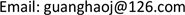1. 引言

1972年，著名数学家与计算机语言逻辑学家D. Scott引入了连续格的概念  。后经过多年研究，具有计算机和数学双重背景的连续格理论被推广到使用更广的连续Domain理论  上，并在一般拓扑学、逻辑学、代数学与计算机逻辑学等基础理论学科的研究上取得了丰硕的研究成果    。拓扑学与连续Domain理论是密不可分的，作为一般拓扑学的延伸，数学家A.V. Arhangel’skii在文献  中首次引入相对拓扑的概念，利用相对的思想研究了一般拓扑学。近年来，而作为连续Domain理论的一种量化推广，徐罗山在文献  中引入相容连续偏序集与定向完备化的概念，从而出现了相容连续Domain理论。受相对拓扑研究思路的启发，作为相容定向集的另一种推广，本文首先利用相对的思想引入相对相容定向集的概念，探讨其基础的性质。然后在相对相容定向完备集基础上给出相对相容小于与相对相容连续偏序集的概念，研究其具有的相关性质给出一些等价刻画。最后证明对于给定的集合T，相对相容双小于同样具有插入性。

2. 预备

1) D是定向集，

2) 存在 p ∈ P 使得 D ⊆ ↓ p ，则称D为P中的相容定向集。

1) ∀ x ∈ P ， ⇓ r x ( ↓ x ∩ K r ( P ) )为相容定向集，

2) ( x = sup ( ↓ x ∩ K r ( P ) ) )。

3. 相对相容定向集

1) D是定向集，

2) 存在 t ∈ T 使得 D ⊆ ↓ t ，则称D为P的相对于T的相容定向集。当T明了时，可简称为相对相容定向集。记 R T ( P ) = { D ⊆ P ：D为P的相对T的相容定向集}。

4. 相对相容连续偏序集

1) x < < r r y ⇒ x ≤ y ；

2) u ≤ x < < r r y ≤ z ⇒ u < < r r z ；

3) x < < r r z ， y < < r r z ，若 x ∨ y 存在，则 x ∨ y < < r r z ；

4) 若0存在且 0 ∈ T ，则 ∀ x ∈ T ，有

5) ⇓ r r x ∈ R T ( P ) 。

2) ∀ D ∈ R T ( P ) ，因P为相对相容定向完备集，故 sup D 存在。若 z ≤ sup D ，有 y ≤ sup D ，又 x < < r r y ，存在 d ∈ D 使得 x ≤ d ，进而 u ≤ d ，所以 u < < r r z 。

3) ，则 sup D 存在且，若 z ≤ sup D ，因为 x < < r r z ， y < < r r z ，故存在 d 1 , d 2 ∈ D 使得 x ≤ d 1 ≤ sup D 且 y ≤ d 2 ≤ sup D ，进而 x ∨ y ≤ sup D ，所以 x ∨ y < < r r z 。

4) ∀ D ∈ R T ( P ) ，若 x ≤ sup D ，则存在 d ∈ D ，使得 x ≤ d 。又0为P上的最小元，即 0 ≤ d ，所以 0 < < r r x 。

5) 任意取 a , b ∈ ⇓ r r x ，则有 a < < r r x 且 b < < r r x 。进而 a ≤ x , b ≤ x ，而 { x } ∈ T ，所以 ⇓ r r x ∈ U ( T ) 。

1) ∀ x ∈ T ， x = sup ⇓ r r x ，

2) ⇓ r r x 为相对T的相容定向集。